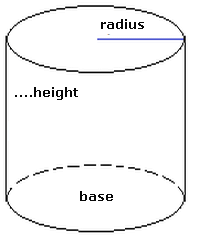### Cylinder

The cylinder is a three-dimensional solid object with two equal circular or elliptical bases and a curved side.
Cylinder elements:
• Radius: is the cylinder base radius;
• Height: perpendicular distance between a horizontal plane of a base and the other;
• Lateral surface: face bounded by the circumferences of the bases;
If the perpendicular straight lines are connected to the circumferences bases, the cylinder is called a right circular cylinder and In this case, also, these straight lines coincide with the height of the cylinder. Otherwise, it is an oblique circular cylinder.
How to calculate the elements of a Cylinder.Was this information helpful? First name: Yes No Not sure